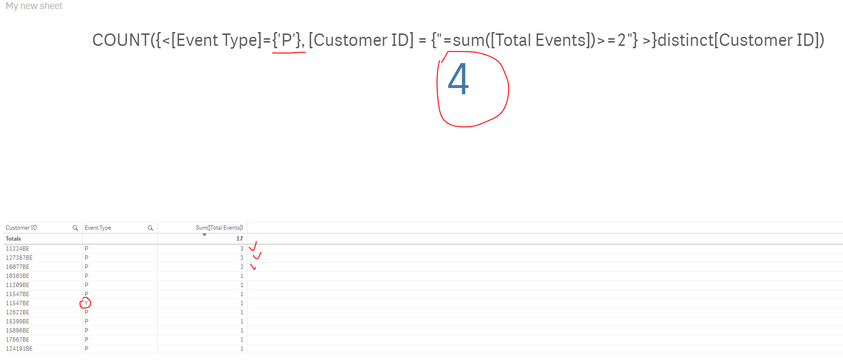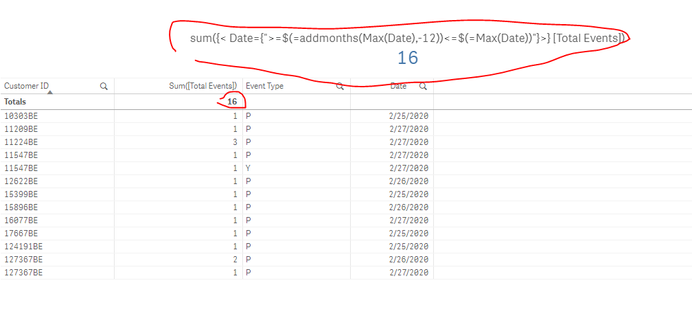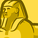# New to Qlik Sense

If you’re new to Qlik Sense, start with this Discussion Board and get up-to-speed quickly.

Announcements
cancel
Showing results for
Did you mean:Contributor III

## Count set analysis does not return expected results

Hi there , I am struggling to understand why my expression gives a wrong result.

I want to calculate customers with event type P whose total events is >= 2. The answer should be 3, but my set analysis giving me 4.

COUNT({<[Event Type]={'P'},
[Customer ID] = {"=sum([Total Events])>=2"}
>}distinct[Customer ID]

The expression seems to ignore the Event Type ='P'.

Thank youLabels (2)

• ### Set Analysis

16 RepliesContributor III
Author

returns me a correct resultContributor III
Author

I tried to fix it by changing the expression to a date range like this

COUNT({<
[Customer ID] = {"=sum({< [Event Type]={'P'},
Date={">=2/25/2020<=2/28/2020"}>}
[Total Events])>=2"}>}
distinct [Customer ID]))

I will get an error .MVP & Luminary

That this expression-part here works showed that the applied date-logic itself and the syntax is generally correct and should be therefore also work within the whole expression. It's difficult to guess why not.

To exclude that yet any formatting-stuff caused it try the following variants (not all together - unless properly commented):

COUNT({<
[Customer ID] = {"=sum({< [Event Type]={'P'},
[Total Events])>=2"}
>}
distinct [Customer ID])

- MarcusContributor III
Author

Hi @marcus_sommer  I very much appreciate your help,  I have tried all of them but they all end up with no results.Contributor III
Author

And my data is very simple, the date column is formate correctly as dateMVP & Luminary

If your other expression-steps from above work it should also work combined. At the moment I don't see an obvious issue. In similar cases I would reduce all possible complexity and looking if it returned the expected values and then adding step by step more conditions/variables/expression-parts to find the point where it breaks respectively it returned unexpected results. In your case you may start with something like this:

COUNT({< Date={">=43886"}>} distinct [Customer ID])
COUNT({< Date={">=43886<=43888"}>} distinct [Customer ID])

- MarcusSpecialist

Hi ,

First try to create a flag in script level for sum of total event is >=2 as below

if sum([Total Events])>=2,'Y',"N') as flag-->script level add this code and also

and then in the expression try this

COUNT({<[Event Type]={'P'},flag={'Y'},